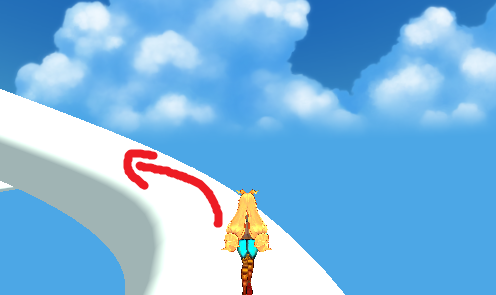# SphereCast help

My problem is this. My player can run around a tube with no problem but when the tube makes a curve she’s always looking forward like this:

The first arrow is where I want her to be looking, at the second is what she is doing because there is no rotation.

The problem starts here:I want her to turn on the curve here looking at the tube, not always forward.

Where is my code

``````        Ray ray;
RaycastHit hit;

// movement code

Vector3 dir = Vector3.zero;
dir.x = Input.GetAxis("Horizontal");
if (dir.sqrMagnitude > 1)
dir.Normalize();

dir *= Time.deltaTime;
transform.Translate(dir * Aspeed);
//myTransform.Rotate(0, Input.acceleration.x * turnSpeed * Time.deltaTime, 0);
//myTransform.Rotate(0, Input.GetAxis("Horizontal") * turnSpeed * Time.deltaTime, 0);

// update surface normal and isGrounded:
ray = new Ray(myTransform.position, -myNormal); // cast ray downwards
if (Physics.Raycast(ray, out hit))
{ // use it to update myNormal and isGrounded
isGrounded = hit.distance <= distGround + deltaGround;
surfaceNormal = hit.normal;
Debug.DrawLine(transform.position, hit.point, Color.blue);
}
else
{
isGrounded = false;
surfaceNormal = Vector3.up; // assume usual ground normal to avoid "falling forever"
}
myNormal = Vector3.Lerp(myNormal, surfaceNormal, lerpSpeed * Time.deltaTime);
Vector3 myForward = Vector3.Cross(myTransform.right, myNormal); // find forward direction with new myNormal
Quaternion targetRot = Quaternion.LookRotation(myForward, myNormal); // align character to the new myNormal while keeping the forward direction
myTransform.rotation = Quaternion.Lerp(myTransform.rotation, targetRot, lerpSpeed * Time.deltaTime);
anim.SetFloat("Speed", moveSpeed);
myTransform.Translate(0, 0, 1f * moveSpeed * Time.deltaTime); // auto move the character
``````

If I use

``````myTransform.Rotate(0, Input.GetAxis("Horizontal") * turnSpeed * Time.deltaTime, 0);
``````

she can rotate but I just want to control the player rotation around the tube, not the curve rotation.

I appreciate if someone can help me.

EDIT:
If I add to my code

``````if (Physics.SphereCast(transform.position, 0.5f, -transform.up, out hit, 5))
{
transform.rotation = Quaternion.Lerp(transform.rotation, Quaternion.LookRotation(Vector3.Cross(transform.right, hit.normal), hit.normal), Time.deltaTime * 5.0f);
}
``````

it does exactly what I want and align the player with the tube, but it makes the player do “small jumps” as if she were walking over spheres.Can anyone help me avoid that?

Use Transform.transformdirection with the character’s transform as the root and the camera’s.

It’ll then take into account the camera direction vector or whereever it is pointing to and turn it to the character’s direction.
It’s not as precise yet, but it’s close enough